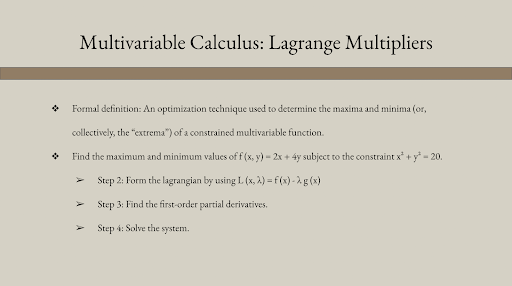top of page
Search

# Multivariable Calculus: Lagrange Multipliers

By Estefania OlaizThe Lagrange Multipliers, otherwise known as undetermined multipliers, are an optimization technique used to determine the maxima and minima (or, collectively, the “extrema”) of a multivariable function. More specifically, they allow us to identify the largest and smallest values of a function subject to constraints.

Lagrange Multipliers prove themselves practical when the variables of a function are made redundant by limiting conditions. For instance, they allow us to resolve concerns like "How do I minimize aluminum usage while ensuring the can will hold 8 ounces of soup?" Or similarly, "How do I maximize my factory's profit given that I only have \$10,000 to invest?" Among their many applications, they are particularly advantageous for business expenditure.

An illustration of their use in regard to the extrema will be demonstrated below. To solve it, we will need to rewrite the constraint, form the lagrangian, find the first-order partial derivatives, and solve the system. Bear in mind that you will need an elementary understanding of partial derivatives to complete the problem with ease.

Problem: Find the maximum and minimum values of f (x, y) = 2x + 4y subject to the constraint x² + y² = 20.

Step 1: Rewrite the constraint.

x² + y² = 20 becomes x² + y² - 20 = 0

Step 2: Form the lagrangian by using L (x, λ) = f (x) - λ g (x).

L (x, y, λ) = (2x + 4y) + λ (x² + y² - 20)

Step 3: Find the first-order partial derivatives.

∂/∂x ((2x +4y) + λ (x² +y² - 20)) = 2 (λx + 1)

∂/∂y ((2x +4y) + λ (x² +y² - 20)) = 2 (λy + 2)

∂/∂λ ((2x +4y) + λ (x² +y² - 20)) = x² +y² - 20

Step 4: Solve the system.

2 (λx + 1) = 0

2 (λy + 2) = 0

x² + y² - 20 = 0

This contains the real solutions of: (x, y) = (-2, -4), (x, y) = (2, 4). Which, in turn, grants us a minimum value of -20, and a maximum value of 20.

You’ve now learned how to find the extrema of a multivariable function by using the Lagrange Multiplier technique, which allows us to solve constrained optimization problems through explicit parametrization. This is highly beneficial if you’re enrolled, or are soon to be enrolled, in a Multivariable Calculus course.

Sources:

1. “Lagrange multiplier,” Wikipedia, May 09, 2022. https://en.wikipedia.org/wiki/Lagrange_multiplier#:~:text=In%20mathematical%20optimization%2C%20the%20method

2. H. Li, “Lagrange Multipliers and their Applications.” [Online]. Available: http://sces.phys.utk.edu/~moreo/mm08/method_HLi.pdf

3. “Calculus III - Lagrange Multipliers,” tutorial.math.lamar.edu. https://tutorial.math.lamar.edu/Classes/CalcIII/LagrangeMultipliers.aspx

4. M. Saeed, “A Gentle Introduction To Method Of Lagrange Multipliers,” Machine Learning Mastery, Aug. 11, 2021. https://machinelearningmastery.com/a-gentle-introduction-to-method-of-lagrange-multipliers/

5. “14.8: Lagrange Multipliers,” Mathematics LibreTexts, Jul. 11, 2016. https://math.libretexts.org/Bookshelves/Calculus/Book%3A_Calculus_(OpenStax)/14%3A_Differentiation_of_Functions_of_Several_Variables/14.8%3A_Lagrange_Multipliers

6. “An Introduction to Lagrange Multipliers,” Slimy.com, 2020. http://www.slimy.com/~steuard/teaching/tutorials/Lagrange.html

See All
bottom of page